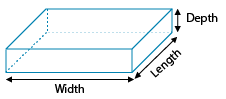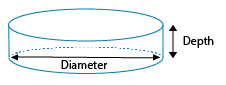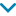Uh Oh! It seems you’re using an Ad blocker!

Since we’ve struggled a lot to makes online calculations for you, we are appealing to you to grant us by disabling the Ad blocker for this domain.# Sand Calculator

Choose the area to fill:Length

Width

Area

Volume

Diameter

Depth / Height

Density

Cost Estimation:Price/unit of mass

Price/unit of volume

Price/ton

Table of Content
 1 What are the Types of Sand? 2 How to Calculate How Much Sand Do I Need (Step-by-Step): 3 How Much Does Sand Cost? 4 What is the density of sand?? 5 How much is a ton of sand?
Get The Widget!

Add Sand Calculator to your website to get the ease of using this calculator directly. Feel hassle-free to account this widget as it is 100% free, simple to use, and you can add it on multiple online platforms.

Available on App

The online sand calculator helps you to estimates how much sand do you need to complete your construction work. Also, this sand cost estimator works out to estimates the total cost of the required sand for your earthwork.

No doubt, estimating manually the required quantity of any construction material is a daunting task. But, no need to worry more, this sand estimator helps in estimating the amount of sand you need to fill a given space with sand. Before doing the required sand calculations, you can choose whether you want to fill the sand for square/rectangle, or circle area.

Well, this context helps you to calculate the amount (quantity) and cost of the sand that you need to complete your civil construction project.

Also, you can try the online mulch calculator that helps you to determine the amount of mulch you required to complete your construction task.

## What Are The Types of Sand?

According to the size of pebbles & their uses, there is more than one type of sand. Selecting the right type matters because they have different applications according to the type. They reclassified into the following categories:

### 20-30 Sand:

It is made up of local natural silica sand having water content low than 0.1%. The parts of this and are uncrushed and in round form.

This type is of moderate size particles and containing coarse. It is not uniform sand.

### Standard Sand:

It is made up of natural silica having the particles in round form of nearly pure quartz. This type of sand is used for the mortars & testing of hydraulic cements.

For the gravel calculations, you can try our online gravel calculator that helps you to do the gravel calculations precisely.

## Formulas For Sand Calculations:

When you are going to find how much sand are required for your project by using this sand estimator, it performs calculations corresponding to the area and volume of the sand required. Here are the formulas that help you to work out!

Area = Length * Width

Volume = Area * Depth

If you already have an idea about the density of the material that you’re using in your project (standard sand is 100 lb/ft³), the sand calculator helps you to find how many tons of sand you will need by using the given formula:

Weight = Volume * Density

Formulas For Finding the cost of sand:

• If you know the price per unit mass: for example “cost per pound” and
• If you have the cost per unit volume: for example “cost per cubic feet”, then the respective formulas might works best for you

Cost = price per unit mass * weight

Cost = price per unit volume * volume

Our sand cost calculator will work out the total cost of the required sand by using these formulas for cost.

## How to Use the Online Sand Calculator:

Our online tool determines the results of your sand calculations accurately. The sand estimator generates the results of the following surfaces:

• Square/Rectangle
• Circle

Just follow the given points for the exact calculations for required sand:

Inputs:

• First of all, you have to choose the area that you want to fill with sand
• Now, choose the option either you want to do calculations according to length and width, area, and volume
• Then, add the values according to the selected option
• Also, enter the for price/unit of mass or price/unit of volume to find the cost of sand (this field is optional)
• Once done, hit the calculate button

Outputs:

The sand estimator estimates:

• Volume of sand in cubic feet (ft³ ) or in different other units such as cubic (millimeters, centimeters, meters, inches, yards) according to given values
• Weight of sand in ton (t) or in different other units including (grams, kilograms, ounces, pounds, stones, short tons, imperial tons)
• This sand cost calculator helps to calculate either the cost of sand for Price/unit of mass or Price/unit of volume

Also, you can try our online roof pitch calculator that helps you to measure the pitch and length of rafters for which you going for construction.

## How Much Sand Do I Need (Step-by-Step):

The sand calculator helps you to determine the volume & weight of sand needed for the construction project. Here we have manual calculations for both.

Example:

The rectangular surface having length 12ft, width 14ft with a depth of 3ft. The density of sand is 100lb/ft3 .Then how much sand do I need for the construction?

Solution:

L = 12ft

W = 14ft

D = 3ft

So, find the area,

A = L × W

A = 12 × 14

A = 168ft2

Now, calculate the volume needed by using the formula:

V = A × D

V = 168 × 3

V = 504ft3

Weight = Volume × Density

Weight = 504ft3× 100lb/ft3

Weight = 504500lb

### How Much Does Sand Cost?

Once you determine the weight & volume of the sand, you can try our sand cost calculator that helps you to find how much you have to pay to buy the sand. Let’s try an example:

Example:

If the price per unit of volume of sand is 5$, then how much does sand cost for the 504ft3 sand? Solution: Cost formula (if the price per unit volume is known): Cost = price per unit volume * volume Cost = 5$ × 504

Cost = $2520 So, if the price per unit mass given, then what you have to do to find cost of the sand. Look at the example: Example 2: The price per unit weight of sand is 12$, then calculate amount of sand needed for 225lb?

Solution:

Cost formula (if the price per unit weight is known)

Cost = price per unit mass * weight

Cost = 12 * 225

Cost = $2700 You can use the above online sand calculator to work out all these calculations instantly. Note: The price of sand typically ranges from 5 to$30 per ton, which depending on the material type. However, natural sand will generally cost less than specialty sand. Screened sand costs about $15 to$20 per cubic yard, and it is highly suitable as a base for paving projects like driveways. Also, you could use sand for sandboxes and hardscaping.

• Typical Range: $322 –$1,148
• National Average: \$734

### What is the density of sand?

Typically, the density of the sand is 100lb/ft3 or 1600 kg/m3.

### How Much Sand Do I Need for My Pool?

Typically, there should be a 2 to 3-inch base of sand under an above ground pool. If the dimensions of the pool area are known, then try sand estimator to figure out how much sand you will need for the base.

### How much is a ton of sand?

0.750 cubic yards or ¾ cu yard or 20cubic feet equals to the one ton of the sand. Sand is relatively damp, since adding water can low or high the density of the sand.

### How much does a yard of sand weigh?

Typically, gravel and sand weighs 2,200 to 2,700 pounds per cubic yard.

### How many bags of sand do i need for pavers?

The recommended depth of sand for laying pavers is typically 1 inch. As 1 inch is equal to 1`/12 of a foot, you need to divide the square area, in square feet, by 12 to figure out the cubic feet of sand needed. For example, a 96 square foot patio paver requires 8 cubic feet of sand because 96/12 = 8.

## Wrapping-it up:

Sand is a key element for concrete, road, glass, and electronics. A massive amount of it is mined for land reclamation projects and beach renourishments programs. Before starting your construction project, you should have to beware of the right quantity of material you need. So, simply try this online sand calculator that helps you to determine the amount and cost of sand required for the completion of your construction task. Builders typically use the online sand cost calculator to estimate the cost of sand required for their projects.

## References:

From the authorized source of Wikipedia : Sand, types & it’s applications

From the site of livescience.com : Composition of sand & much more

From the source of waupasanad: How much materialdo I need for construction